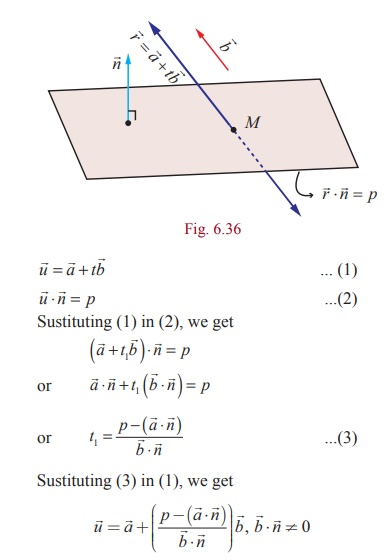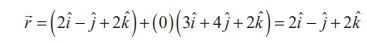Home | | Maths 12th Std | Meeting Point of a Line and a Plane

# Meeting Point of a Line and a Plane

The position vector of the point of intersection of the straight line and the plane

Meeting Point of a Line and a Plane

### Theorem 6.23

The position vector of the point of intersection of the straight lineand the plane### Proof

Letbe the equation of the given line which is not parallel to the given plane whose equation isLetbe the position vector of the meeting point of the line with the plane. Thensatisfies bothand= p for some value of t , say t1. So, We getExample 6.56

Find the coordinates of the point where the straight lineintersects the plane x y + z − 5 = 0 .

SolutionThe vector form of the given plane isWe know that the position vector of the point of intersection of the lineand the planeTherefore,the position vector of the point of intersection of the given line and the given plane isThat is, the given straight line intersects the plane at the point (2, −1, 2 ).

Aliter

The Cartesian equation of the given straight line is(say)

We know that any point on the given straight line is of the form (3t + 2,4 t-1,2t+ 2) . If the given line and the plane intersects, then this point lies on the given pane x-y+z-5=0.

So, (3t + 2) - (4t-1) + (2t+ 2) - 5 = 0 t = 0.

Therefore, the given line intersects the given plane at the point  (2,-1, 2)

Tags : Definition, Theorem, Proof, Solved Example Problems, Solution , 12th Mathematics : UNIT 6 : Applications of Vector Algebra
Study Material, Lecturing Notes, Assignment, Reference, Wiki description explanation, brief detail
12th Mathematics : UNIT 6 : Applications of Vector Algebra : Meeting Point of a Line and a Plane | Definition, Theorem, Proof, Solved Example Problems, Solution